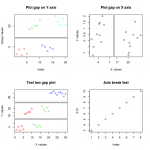Categories

## Two ways to save a plot in png…

Two ways to save a plot in png.

dev.copy() saves the plot with transparent background while savePlot() with white background.
See the difference.

```dev.copy(device = png, file = "devcopy.png")
dev.off()
```
```savePlot(file = "saveplot.png")
```

Categories

## Using discontinuous axis or breaks may not…

Using discontinuous axis or “breaks” may not be a good idea to visualize data.
Instead, use two plots, one with the whole data and another for zoomed data.
However, if the situation is not allowed, there is an R package to do it.
The package plotrix can put breaks or gaps on the axes.Categories

## How to use eval Here is an example…

How to use eval()?
Here is an example.

```test.dframe <- data.frame(x = 1:10, y = rnorm(10))
addSmooth <- function(data, smooth = TRUE) {
require(ggplot2)
p <- "ggplot(data = data, aes(x = x, y = y))"
p.line <- "geom_line()"
p.smooth <- "stat_smooth()"
if (smooth == TRUE) {
p.all <- paste(p, p.line, p.smooth, sep = " + ")
} else {
p.all <- paste(p, p.line, sep = " + ")
}.
eval(parse(text = p.all))
}

Created by Pretty R at inside-R.org

Categories

## checkUsage in the codetools package examines the function…

checkUsage() in the codetools package examines the function and report possible problems.

R functions look for a global variable if the variable is not found in the scope. If a function accidentally has an “orphan” variable which is not passed on to the function but has the same name as one of the global variables, the function will use the value of the global variable. It is a hard to find bug. checkUsage() will find such cases and report.

Categories

## Multiple plots with different titles using ggplot lapply…

Multiple plots with different titles using ggplot, lapply, and do.call.

```parameters = data.frame(p1=letters[1:5], p2=round(rnorm(5),2))
l = replicate(5, data.frame(x=1:10, y=rnorm(10)), simplify=FALSE)
names(l) = do.call(paste, c(parameters, sep=","))

plot_one = function(x)
ggplot(data = l[[x]]) + geom_path(aes(x, y)) +
opts(title = x)

plots = lapply(names(l), plot_one)

do.call(gridExtra::grid.arrange, plots)```

Created by Pretty R at inside-R.org

http://stackoverflow.com/questions/10726470/ggplot-over-many-data-frames-changing-titles